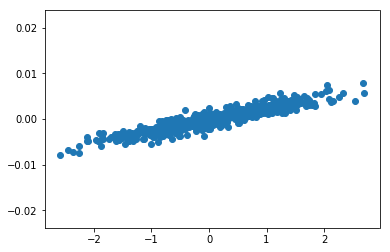# AI For Trading:Transfer Coefficient (73)

## Transfer Coefficient(传递系数)

Recall that we prefer to control for risk within the Alpha factors,rather than waiting for their risk model optimizer to do it completely for us.

This is why we make the Alpha vector dollar neutral and often also sector neutral. The reason we wish to control for risk early on within the Alpha vector itself is so that after the optimization, the portfolio weights are not too different from the original Alpha vector.

It's natural for you to wonder why is it an issue at the portfolio weights are nothing like the original Alpha vector?

To understand this, remember that we're evaluating each Alpha factor in isolation of other Alpha vectors.

We evaluate the Alpha factor to see if it may be a good candidate to help improve the performance of a portfolio.
If after optimization, the risk model significantly changes the weights for stock so that they no longer follow the weights chosen by the Alpha vector, then we can't expect the performance of the Alpha to carry over into the portfolio.

As the example, imagine if an Alpha vector gives most positive weight to stock ABC.
But after the risk model neutralizes various common risks, the final portfolio weights end up shorting stock ABC, then the signal from the Alpha vector which essentially says,"Buy stock ABC" is no longer being followed.

This means that the performance that we originally hoped to gain from using this Alpha vector may not carry over or transfer to the portfolio itself.

Remember that the Alpha vector is an expression of expected returns. If the portfolio weights after every optimization are very different from the original Alpha factors, then the portfolio itself may not be capturing the information expressed by those Alpha vectors.

To see how closely the optimized portfolio weights still match the original Alpha vector, we can calculate something called the transfer coefficient.

The transfer coefficient measures how closely the optimized portfolio weights match the original Alpha vector. To get the transfer coefficient,we need to use portfolio optimization that includes a risk factor model.

We will learn about the portfolio optimization step in a later lesson. For now, let's just assume that we have a portfolio optimizer that outputs portfolio weights. We start with our Alpha vector, pass it through the portfolio optimization and get portfolio weights. The transfer coefficient is the correlation between the Alpha vector and the resulting portfolio weights.

A high transfer coefficient is a good sign that the Alpha vector has survived the portfolio optimizers risk neutralization.
The transfer coefficient is also a useful measure for another desired attribute of an Alpha factor independence from risk factors.

Ideally, we would like to find Alpha factors that are independent of the common risk factors. If Alpha factors were too correlated with the risk factors, then they would also be neutralized by the risk model.

## Transfer Coefficient Coding Exercise

%matplotlib inline
import matplotlib.pyplot as plt
import numpy as np
from scipy.stats.stats import pearsonr
from scipy.stats.stats import spearmanr
num_stocks = 500
np.random.seed(201808)
def standardize_alpha(alpha_vector):
return(alpha_vector - np.mean(alpha_vector))/np.sum(np.abs(alpha_vector))

## Simulate an alpha vector for a single time period

#make up data for an alpha vector for a single time period
def simulate_alpha_vector():
alpha_vector = np.random.normal(size=num_stocks)
return alpha_vector
alpha_vector = simulate_alpha_vector()

print(alpha_vector[0:5])
[-0.5392397  -0.79946616 -0.14905277  1.22239826  0.46789588]

## Simulate optimized portfolio weights

You'll learn about optimization using a risk model and constraints in a later lesson. For, now, we'll simulate portfolio weight optimization by adding some adjustments to the original alpha vector, and pretending these adjusted values are the portfolio weights that are output by an optimizer.

def simulate_optimized_weights(alpha_vector):
standard_alpha_vector = standardize_alpha(alpha_vector)
#add some noise that simulates optimization adjusting the weights.
optimized_weights = standard_alpha_vector + np.random.normal(scale=0.001, size=num_stocks)
return optimized_weights
optimized_weights = simulate_optimized_weights(alpha_vector)

## Visualize alpha vector versus optimized weights

plt.scatter(alpha_vector,optimized_weights);def calculate_transfer_coefficient(alpha_vector, optimized_weights):
#TODO calculate the transfer coefficient
transfer_coefficient, pvalue = pearsonr(alpha_vector, optimized_weights)
return transfer_coefficient
transfer_coefficient = calculate_transfer_coefficient(alpha_vector, optimized_weights)
print(f"The transfer coefficient is {transfer_coefficient:.4f}") 
The transfer coefficient is 0.9291